# Realisation problem for the space of knots in the 3-sphere

 Importance: Medium ✭✭
 Author(s): Budney, R
 Subject: Topology
 Keywords: knot space symmetry
 Recomm. for undergrads: no
 Posted by: rybu on: November 7th, 2009
Problem   Given a linkin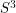, let the symmetry group ofbe denoted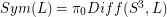ie: isotopy classes of diffeomorphisms ofwhich preserve, where the isotopies are also required to preserve.

Now letbe a hyperbolic link. Assumehas the further Brunnian' property that there exists a component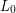ofsuch thatis the unlink. Letbe the subgroup of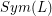consisting of diffeomorphisms ofwhich preservetogether with its orientation, and which preserve the orientation of.

There is a representation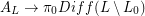given by restricting the diffeomorphism to the. It's known thatis always a cyclic group. And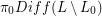is a signed symmetric group -- the wreath product of a symmetric group with.

Problem: What representations can be obtained?

An answer to this problem would give a closed form' description of the homotopy type of the space of smooth embeddings of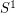in. This is the space of embeddings in the Whitney Topology, or-uniform topology for any.

`Closed form' means that every component of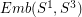would have the description as an iterated fiber bundle over certain well-known spaces, where the fibers are inductively well-known spaces, and the monodromy would be controlled rather explicitly by this list of representations.

Peripherally related are various other realization problems for-manifolds. For example, Sadayoshi Kojima proved that one can realize any finite group as the group of isometries of a hyperbolic-manifold.

## Bibliography

*[B] Budney, R. Topology of spaces of knots in dimension 3, to appear in Proc. Lond. Math. Soc.

[B2] Budney, R. A family of embedding spaces. Geometry and Topology Monographs 13 (2007).

[K] Kojima, S., Isometry transformations of hyperbolic-manifolds. Topology Appl. 29 (1988), no. 3, 297--307.

* indicates original appearance(s) of problem.